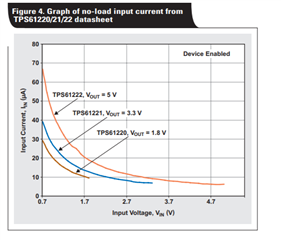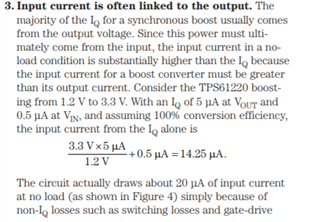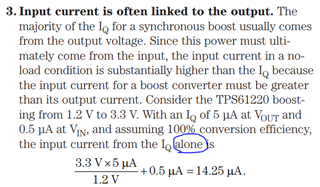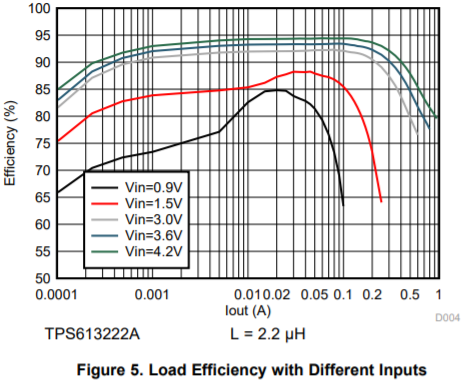If you have a related question, please click the "Ask a related question" button in the top right corner. The newly created question will be automatically linked to this question.

Part Number: TPS613221AEVM

Hello. The datasheet of TPS613221ADBVR select - Iq in VOUT = 6.5uA. What did it mean? What is the input (Quiescent) current if: Vout=3.3V, Iout=0mA, Vinput=1.5V?

• Hi Alex,

e2e.ti.com/.../IQ-_2D00_-What-it-is_2C00_-what-it-isn_1920_t_2C00_-and-how-to-use-it.pdf

We measure VOUT IQ through exert an external source on VOUT to make it no switching, VIN is normal, then measure the current into VOUT pin.

-Wenhao

•Those I understand correctly that the input current at Vin = 1.5V and no load will be about 15 μA? ((3.3*6.5)/1.5)+0.5=14.8uA

• Hi Alex,

The input current at no load will be much higher than that number because the device is switching.

The calculation is for IQ alone, and assume efficiency to be 100%.-Wenhao

• `I'm just trying to understand what current will be from the 1.5V battery if the device is in sleep mode (while the load current will be about 5uA) if I use TPS613221ADBVR`
• If I understand correctly current from battery without load  is - ( (3.3*IqVOUT) / Efficiency  / Vin )+IqVIN

`Did I understand correctly?`
• Hi Alex,

You can take the efficiency curve in the datasheet and calculate input current at desired operating point. This can more accurately calculate input current which also takes switching loss into account.

For 1.5Vin, 100uA load, TPS613222A has about 75% efficiency. Therefore the input current is 5*100/1.5/0.75=444.44uA.-Wenhao